## what is the equation of the line passing through the point (2,-1) with a slope of -3

Question

what is the equation of the line passing through the point (2,-1) with a slope of -3

in progress 0
3 weeks 2021-09-03T13:54:46+00:00 1 Answers 0 views 0

1. Note: this answer assumes that the equation of the line must be in slope-intercept form.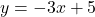Explanation:

When you need to write an equation and know its slope and a point it intersects, you can use the point slope formula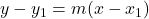first. In order to write an equation using this formula, the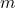,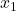, and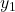must be substituted in for real values.

Therepresents the slope, so write the slope of this equation, -3, in its place. Theandrepresent the x and y values of a point the line intersects. We know that the line must intersect the point (2, -1). Write 2 in the place of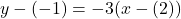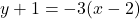Now, to put it in slope-intercept or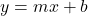form, just expand the right side and isolate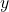: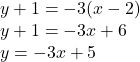Therefore,is the answer.